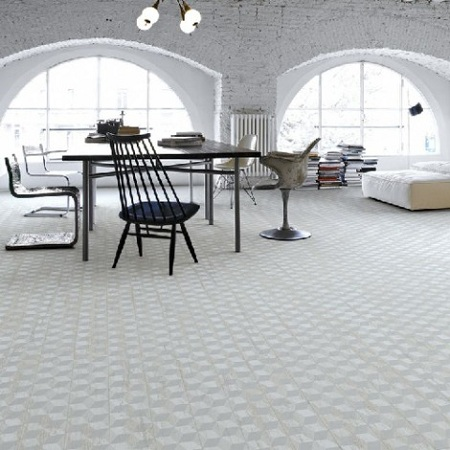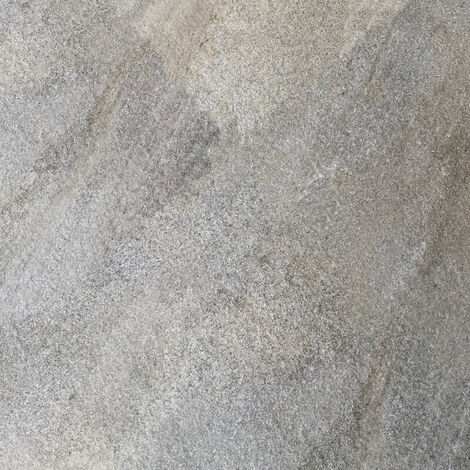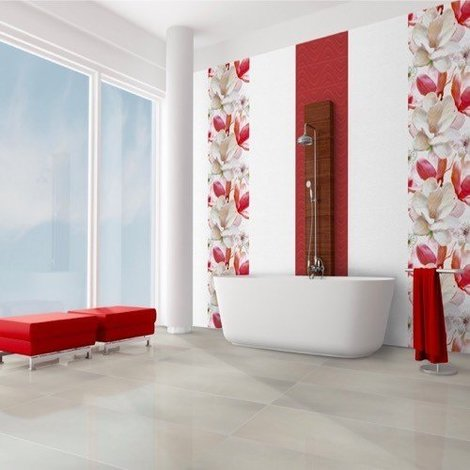# How to calculate how many tiles you needGuide written by:

Pauline, Self-taught handyman, Leeds

97 guides

Before you set about laying tiles, you need to figure out how many you need to order for your project. To do so, you'll need to think about the format and layout of your tiles, as well as the size of the room. Read on to find out how best to calculate how many tiles you need!

## Important features

• Calculating area
• Factoring in wastage
• Calculating skirting board tiles

## Calculating tile quantities: 4 factors to bear in mindWhen it comes to estimating the number of tiles you need, there are four different factors to consider:

1. The overall dimensions of the tiles: length multiplied by width (L x W).
2. The layout of your tiles: straight installation (+ 5%) or diagonal installation (+ 10%).
3. The surface area in m²: calculate the area according to the shape of the room (L x W for a rectangular room).
4. Factor in an extra 15%: in case of mistakes or breakage.

In addition to these four factors, you might want to think about the thickness of the tile grout. However, given how little influence this will have on the size of the tiled surface overall and the fact that you will be purchasing more tiles than you actually need (to account for cutting tiles, breakage, mistakes and replacement tiles), you usually don't have to factor this into your calculations.

### How many tiles per m²

Here is the correlation between some standard tile dimensions and the number of tiles you'll need per metre squared (before factoring in extra tiles for wastage):

 Tiles per m² Tile dimensions Number of tiles per m² 5 x 5 cm 400 tiles 10 x 10 cm 100 tiles 15 x 15 cm 45 tiles 20 x 20 cm 25 tiles 20 x 30 cm 17 tiles 30 x 30 cm 12 tiles 60 x 60 cm 3 tiles

## Why order more tiles than you need?Calculated the number of tiles you need to tile your kitchen, bathroom or entrance? Now you need to factor in any potential accidents that could occur such as dropping tiles, cutting them incorrectly or making the wrong calculations in the first place. To do so, it's recommended to order around 15% more tiles than your original calculations.

Furthermore, if part of the tiled surface is to be tiled diagonally (in a walk-in shower, for example), you will need more tiles. In this case, multiply the amount of tiles you think you need by 1.5; for example, if you need 30 small tiles to cover your surface, get 45.

With a few extra tiles, you can rest easy knowing you'll be able to finish your project without the risk of any setbacks such as your tiles going out of stock. It is also advisable to buy all your tiles at once in order to avoid any differences in shade or size, which would be very frustrating when it comes to installation.

When you buy your tiles be sure to check the returns policy, especially if you buy during a sale. If your tiles are on sale, be sure to check whether or not you can return them. This way, you'll know in advance if you can get a refund for any spare tiles.

## How to measure an area to calculate tile quantity

Whether you're measuring a floor or a wall, your calculations will depend on the shape of the surface you want to tile. The easiest area to calculate is a rectangular room with right angles (both floor and ceiling). However, you might need to measure the area of a room with a trapezoid or even triangular-shaped floor. Don't worry: there's nothing too complicated about it, provided you follow a few basic formulas!

### Calculating the area of a rectangular roomIn order to calculate the area of a rectangular room you will simply have to multiply the length of the room in metres by its width (L x W).  This will provide you with the area of the room in metres squared (m²). For example, if your bathroom is 3 metres long and 4 metres wide, use the following calculation to find out the area of the floor: 3 x 4 = 12. For the floor of this room, you'd therefore need 12 metres of tiles.

### Calculating the area of a trapezoid-shaped roomTo find the area of a trapezoid-shaped room, you'll need to add together the length of the parallel sides of the room and multiply this number by the height of the room before dividing the result by two. Here is the formula to use:

• Area = [(length 1 + length 2) x height] / 2

For example, if your laundry room measures 4.5 metres by 5.3 metres and has a height of 2.5 metres, use the following calculation:

• [(4.5 + 5.3) x 2.5] / 2 = (9.8 x 2.5) / 2 = 12.25 m²

### How to calculate the area of a triangular room

To do so, you will need to multiply the length of the base of the triangle (L) by the height of the triangle (H) (the sides that form an angle with the base). The formula is as follows:

• Area = B x H

For example, for a triangular bathroom with a length of 5 metres and a width of 3 metres, use the following calculation:

• 5 x 3 = 15 m²

To calculate the area of an awkwardly shaped room, a good tip is to divide the surface into three areas then add these figures together to get the total area.

Looking to calculate the size of a wall? The calculations are the same but you will have to factor in any empty spaces in the walls (doors, windows, fixed furniture, etc.).

## Formulas to work out how many tiles you need

To find out how many tiles you need, your calculations should take into account any particular features of the room.

### How to buy tiles: practical examplesFor a bathroom, for example, it is generally recommended to get 7% more tiles than your total area. Need to cover a surface measuring 30 m²? If you go for tiles measuring 60 x 60 cm, you'll need 90 to cover 30 m².

If your tiles will be installed straight (meaning the edges will be parallel to the wall) you should factor in at least an extra 5% for wastage. In this case, you will need to multiply the amount of tiles you need for your area by 1.05 to calculate for 5% more than your original estimate. For an extra 6%, multiply your estimate by 1.06; for 7%, multiply your estimate by 1.07, and so on. This will help you to calculate the total amount of tiles you need, wastage included.

If you are tiling diagonally (for example, if your walls are not entirely straight), factor in an extra 10%. In this case, you'll then have to multiply your original estimate by 1.1.

### How to calculate tiles for a skirting board

To find out how many tiles you need to form a skirting board, use the following basic calculation:

• length of wall minus length of empty spaces or obstacles

To calculate the number of tiles you need per metre of skirting board, divide the length of the tiles by 1 metre (100 cm):

• 100 cm / x cm (x = length of tile in cm)

Here is the ratio of the number of tiles per metre for different tile formats:

 Guidelines for 1 metre of tile Length of tile Number of tiles per metre 5 cm 20 tiles 10 cm 10 tiles 30 cm 3.5 tiles

Before you start buying tiles, take the time to calculate exactly how many tiles you need for your job. To do so, create a floor plan of the room and its measurements alongside the corresponding calculations. You can then take note of how many extra tiles you need to buy.

Guide written by:

Pauline, Self-taught handyman, Leeds, 97 guidesWith a handyman-father, I grew up with the soft sound of the sander and hammer on weekends. I am both manual and cerebral (yes, it is possible.), I learned the basics of DIY and the customization of furniture because I was passionate. The salvage mentality is a true way of life that allowed me to know how to use all the tools and products needed to give something a second life, from sander to varnish. I have two favorite activities: the transformation of old furniture and decoration tips. I am always ready to lend a helping hand to revamp a table or to restore a mirror that was intended for the trash that will become a friend’s centerpiece. I’m convinced that it’s possible to reinvent an interior by small, regular modifications, I constantly research low-cost, test ideas.